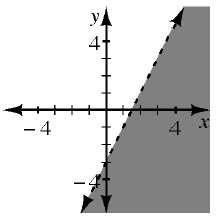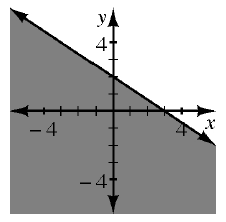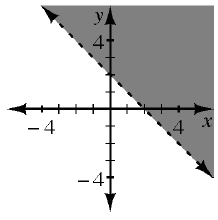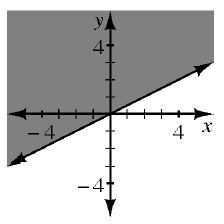### Home > AC > Chapter 9 > Lesson 9.3.1 > Problem9-62

9-62.

Match each graph below with the correct inequality.

1. $y>-x+2$

Which graph represents the line $y=-x+2$?

Graph 3

1. $y<2x-3$

Follow the steps in part (a).

Graph 1

1. $y\ge\frac{1}{2}x$

Follow the steps in part (a).

1. $y\le-\frac{2}{3}x+2$

Follow the steps in part (a).

1.1.1.1.